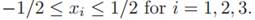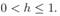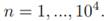# Explore some of the properties of density estimation in the following way. (a) Write a program to generate points according to a uniform distribution in a unit cube,  Generate 104 such points. (b) Write a program to estimate the density at the origin based on your 104 points as a function of the size of a cubical window function of size h. Plot your estimate as a function of h, for (c) Evaluate the density at the origin using n of your points and the volume of a cube window which just encloses n points. Plot your estimate as a function of (d) Write a program to generate 104 points from a spherical Gaussian density (with Σ = I) centered on the origin. Repeat (b) & (c) with your Gaussian data. (e) Discuss any qualitative differences between the functional dependencies of your estimation results for the uniform and Gaussian densities.

Question
1 views

Explore some of the properties of density estimation in the following way.

(a) Write a program to generate points according to a uniform distribution in a unit cube,Generate 104 such points.

(b) Write a program to estimate the density at the origin based on your 104 points as a function of the size of a cubical window function of size h. Plot your estimate as a function of h, for(c) Evaluate the density at the origin using n of your points and the volume of a cube window which just encloses n points. Plot your estimate as a function of(d) Write a program to generate 104 points from a spherical Gaussian density (with Σ = I) centered on the origin. Repeat (b) & (c) with your Gaussian data.

(e) Discuss any qualitative differences between the functional dependencies of your estimation results for the uniform and Gaussian densities.

### This question hasn't been answered yet.